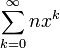An adder is a digital circuit designed to perform integer addition in the Arithmetic Logic Unit on board a computer. These circuits are fundamental to the operation of a computer and have an analog in traditional pencil-and-paper addition.

Integers can be represented by the sum of a series from 0 to infinity.$\sum_{k=0}^\infty nx^k$
• Where n is an integer from zero to (base - 1)
• Where x is an integer equal to the base value.

Example, base x = 10, then$123 = (3)(10)^0 + (2)(10)^1 + (1)(10)^2 + (0)(10)^3 + ... + (0)(10)^\infty$

An adder performs a binary operation (two operands) where the n of one power in integer A is added to the n of the same power in integer B. This produces two outputs, a sum, and a carry. The carry is always equal to (sum - (base - 1)). The carry is then added to the sum of the next power's sum, and so on. This represents what is known as a full adder. Each addition operation performed is known as a half adder. Chain a number of half adders together, and a full adder emerges.Some content on this page may previously have appeared on Citizendium.